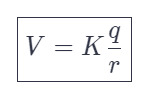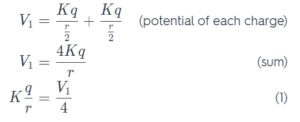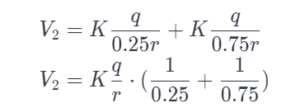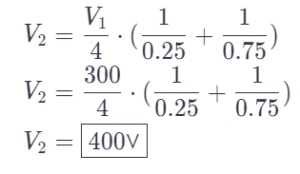# What is the potential at a point that is 25% of the way from one particle to the other?

We thoroughly check each answer to a question to provide you with the most correct answers. Found a mistake? Let us know about it through the REPORT button at the bottom of the page.

The electric potential at a point that is halfway between two identical charged particles is 300 V. What is the potential at a point that is 25% of the way from one particle to the other?

## Explanation

We can start a solution with expression for the potential of a point charge:In the middle between two charges potential is:Potential at a point that is 25% of the wat from one particle to the other is:If we substitute in the previous equation we get: